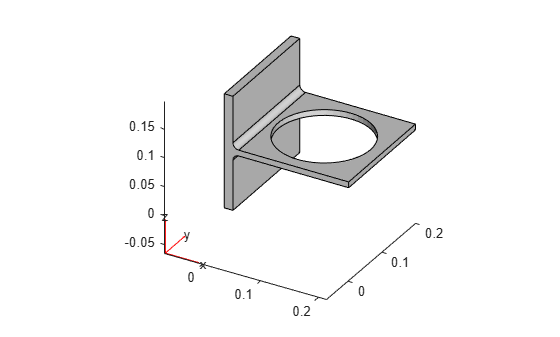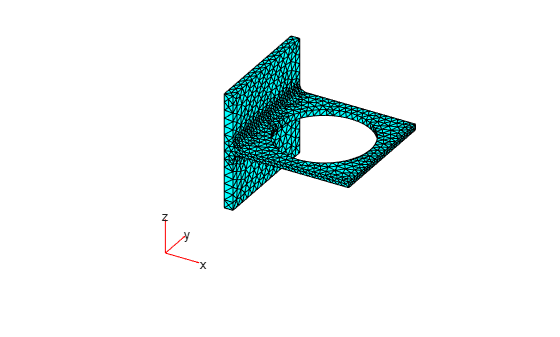# volume

Volume of 3-D mesh elements

## Syntax

``V = volume(mesh)``
``[V,VE] = volume(mesh)``
``V = volume(mesh,elements)``

## Description

example

````V = volume(mesh)` returns the volume `V` of the entire mesh.```

example

````[V,VE] = volume(mesh)` also returns a row vector `VE` containing volumes of each individual element of the mesh.```

example

````V = volume(mesh,elements)` returns the combined volume of the specified elements of the mesh.```

## Examples

collapse all

Generate a 3-D mesh and find its volume.

Create a PDE model.

`model = createpde;`

Import and plot the geometry.

```importGeometry(model,"BracketWithHole.stl"); pdegplot(model)```Generate a mesh and plot it.

```mesh = generateMesh(model); figure pdemesh(model)```Compute the volume of the entire mesh.

`mv = volume(mesh)`
```mv = 8.0244e-04 ```

Generate a 3-D mesh and find the volume of each element.

Create a PDE model.

`model = createpde;`

Import and plot the geometry.

```importGeometry(model,"BracketWithHole.stl"); pdegplot(model)```Generate a mesh and plot it.

```mesh = generateMesh(model); figure pdemesh(model)```Compute the volume of the entire mesh and the volume of each individual element of the mesh. Display the volumes of the first 5 elements.

```[va,vi] = volume(mesh); vi(1:5)```
```ans = 1×5 10-6 × 0.0682 0.2149 0.4491 0.0957 0.4851 ```

Find the combined volume of a group of elements of a 3-D mesh.

Create a PDE model.

`model = createpde;`

Import and plot the geometry.

```importGeometry(model,"BracketWithHole.stl"); pdegplot(model)```Generate a mesh and plot it.

```mesh = generateMesh(model); figure pdemesh(model)```Evaluate the shape quality of the mesh elements and find the elements with the quality values less than 0.5.

```Q = meshQuality(mesh); elemIDs = find(Q < 0.5);```

Compute the total volume of these elements.

`mv05 = volume(mesh,elemIDs)`
```mv05 = 7.9638e-07 ```

Find how much of the total mesh volume belongs to these elements. Return the result as a percentage.

`mv05_percent = mv05/volume(mesh)*100`
```mv05_percent = 0.0992 ```

## Input Arguments

collapse all

Mesh object, specified as the `Mesh` property of a `PDEModel` object or as the output of `generateMesh`.

Example: `model.Mesh`

Element IDs, specified as a positive integer or a matrix of positive integers.

Example: `[10 68 81 97 113 130 136 164]`

## Output Arguments

collapse all

Volume of the entire mesh or the combined volume of the specified elements of the mesh, returned as a positive number.

Volume of individual elements, returned as a row vector of positive numbers.

## Version History

Introduced in R2018a